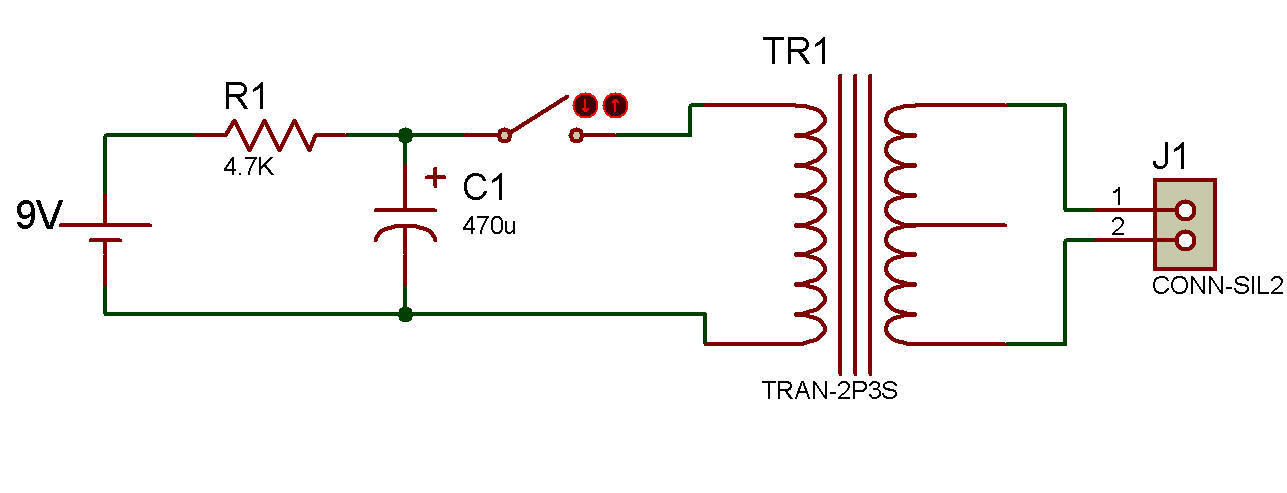Capacitor discharge high voltage generatorThis project shows how single pulses of high voltage are generated when the charged Capacitor is suddenly discharged through the low-voltage windings of the Transformer.

This action is used in capacitor-discharge automotible ignition systems. The operation of the circuit may be simple, but the concepts involved are very basic and therefore important to the understanding of many other more complicated circuits.

Energy is stored up in the 470uF Capacitor by the battery supplying many excess electrons to the negative electrode, and at the same time drawing from the positive electrode the same number of electrons so that it is deficient in electrons. Because of the current limiting action of the 4.7K Resistor, it requires at least 12 seconds for the Capacitor to receive the 9V charge from the Battery.

The amount of charge in a Capacitor can be indicated by either the voltage across the capacitor or (more accurately) by the quantity of electrons displaced in one of the electrodes of the capacitor. The quantity of electrons is measured in "coulombs", where one coulomb is a quantity of 6,280,000,000,000,000,000 electrons (6.28 X 1018 in scientific notation).

To determine the charge (Q) you multiply the capacitance (C) times the voltage (E) across the capacitor (Q = C X E).

For the 470 uF at 9V this is calculated as:

Q = C X E = 470 X 10-6 X 9 = 4.23 X 10-3 coulombs

When the above amount of electrons is passed through the Transformer winding in a very short time, it induces a high voltage in the second (secondary) winding. If you have a neon voltage tester you can connect it across the output to indicate the presence of 90V or more. This is possible because the neon lamp requires at least this voltage before it will ionize and give off light.

Recherche personnalisée